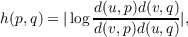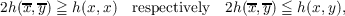#### Vol. 25, No. 3, 1968

 Download this articleFor screen For printingRecent Issues Vol. 325: 1 Vol. 324: 1  2 Vol. 323: 1  2 Vol. 322: 1  2 Vol. 321: 1  2 Vol. 320: 1  2 Vol. 319: 1  2 Vol. 318: 1  2Online Archive Volume: Issue:The Journal Subscriptions Editorial Board Officers Contacts Submission Guidelines Submission Form Policies for Authors ISSN: 1945-5844 (e-only) ISSN: 0030-8730 (print) Special Issues Author Index To Appear Other MSP Journals
Curvature in Hilbert geometries. II

### Paul Joseph Kelly and Ernst Gabor Straus

Vol. 25 (1968), No. 3, 549–552
##### Abstract

The interior of a closed convex curve C in the Euclidean plane can be given a Hilbert metric, which is preserved by projective mappings. Let p, q be points interior to C and let u, v be the points of intersection of the line pq with C. The Hilbert distance h(p,q) is defined bywhere d(x,y) denotes Euclidean distance. If C contains at most one line segment then h(p,q) is a proper metric and the metric lines are the open chords of C carried by the Euclidean lines. Following Busemann [1, p. 237], we define the (qualitative) curvature at a point p as positive or negative if there exists a neighborhood U of p such that for every x,y U we havewhere x y are the Hilbert midpoints of p and x and of p and y respectively.

In an earlier paper  we proved that any point p at which the sign of the curvature is determined is a projective center of C; that is, there exists a projective transformation which maps p into an affine center of the image of C. We also stated the conjecture that a Hilbert geometry has no point of positive curvature. It is the purpose of this paper to prove that conjecture.

Primary: 52.50
##### Milestones